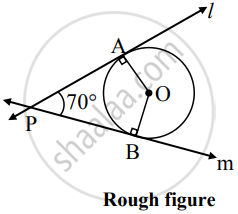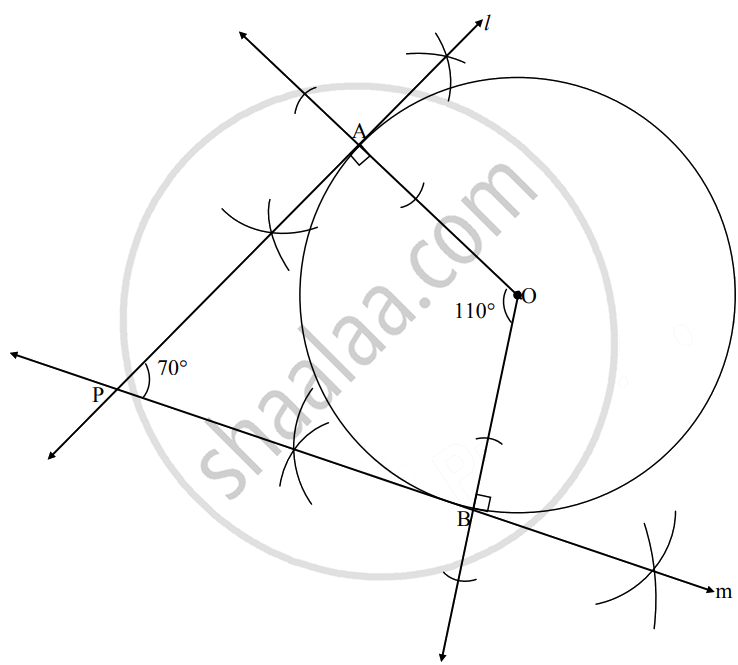# Draw a circle with center O and radius 2.8 cm. Take point P in the exterior of a circle such that tangents PA and PB drawn from point P make an angle ∠APB of measure 70° - Geometry

Diagram

Draw a circle with center O and radius 2.8 cm. Take point P in the exterior of a circle such that tangents PA and PB drawn from point P make an angle ∠APB of measure 70°

#### SolutionAnalysis:

As shown in the figure,

In ▢AOBP,

∠A = ∠B = 90°    ......[Tangent theorem]

∠P = 70°     ......[Given]

∴ ∠O = 110°     .......[Remaning angle of ▢AOBC]Steps of constructions:

1. With center O, draw a circle of radius 2.8 cm.
2. Take any point A on the circle and draw ray OA.
3. Draw line l ⊥ ray OA at point A.
4. Draw ray OB such that ∠AOB = 110°.
5. Point B must be on the circle.
6. Draw line m ⊥ ray OB at point B.
l and m are the required tangents.
Concept: Construction of a Tangent to the Circle at a Point on the Circle
Is there an error in this question or solution?

Share